## Dante Camargo

ID:1266
First Name:Dante
Last Name:Camargo
Last Change:2023-05-07
Number of Files:8 (201st most prolific)

 Formo 9.74/2KX   (details) 49/50 ENG ESP   324KB/23-32KBHelps you browse, create, store, evaluate, delete, move, copy, modify, rename, arrange, backup, and share formulas (equations), all within an easy and friendly user environment. Includes auto-help and was made in User RPL. Version 2KX is animated and is optimized for the 49g+ and 50g; version 9.74 is optimized for the 49G. By Dante Camargo. 2006/11/24

 HP Prime Skins 7.0   (details) Prime ENG   6189KB New set of skins for the Prime emulator. Includes 19 different horizontal and vertical versions in various sizes. By Dante Camargo. 2023/05/07

 KOOL STACK   (details) 49/50 ENG ESP   5KB/2KB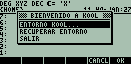Allows you to change the environment of the stack. This program should work in all HP 49G ROM's. By Dante Camargo. 2001/01/29

 LibKit 1.1   (details) 49/50 ENG ESP   135KB/9KBAllows one to create, install, uninstall, and extract libraries, as well as to change a library's ID number. By Dante Camargo. 2001/04/12

 Linsy 2000 1.0   (details) 49/50 ENG ESP   4KB/2KB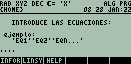Asks you to input equations (even fractions), as long as you enter the same number of equations as there are variables. Then it shows you the equations again, in order for you to check them out and see if you typed them right. The program can then either put the results on the stack or send you to the matrix editor so you can fix the equations. By Dante Camargo. 2000/12/26

 Recta 2000 1.0   (details) 49/50 ENG ESP   5KB/5KB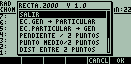Asks you for an input (decimals or fractions) and calculates the center of a rectangle, switches from general to particular equation of a rectangle and vice versa, finds the slope of a rectangle, given 2 points, finds the distance between 2 points, finds the shorter distance between a rectangle and a point, finds the equation of a rectangle, given a point and the slope, and finds the equation between 2 points. By Dante Camargo. 2000/12/26

 SLK (Straight Line Kit) 1.0   (details) 49/50 ENG ESP   364KB/77-82KB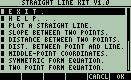SLK (Straight Line Kit) is a User RPL program specially focused on the straight line. It helps you to find the graph of a straight line, the slope between two points, the distance between two points, the middle-point coordinates, the perpendicular distance between a point and a straight line, the equation of a straight line that goes through a point with a slope, the equation of a straight line between two points, the (x) & (y) intercepts of a straight line, and the intersection point of a system of 2 linear equations, plus it will transform linear equations back and forth between standard and slope-intercept forms. Also includes a help section. Works on the 50g and the 49g+. By Dante Camargo. 2013/12/22

 SLK (Straight Line Kit) 4.1b   (details) Prime ENG ESP   1050KB/280KB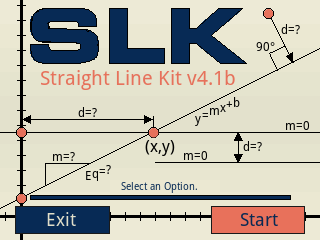Helps you to find the distance, the slope and the equation between two points, the perpendicular distance between a point and a straight line, collinearity of three points (the middle-point and the end-point coordinates), the equation of a straight line that goes through a point with a slope, transforms linear equations back and forth between standard and slope-intercept forms, generates the coordinate representation of a straight line, determines whether two straight lines are parallel or perpendicular, finds the (x) & (y) intercepts of a straight line, finds the intersection point of 2 straight lines, transforms a non-standard form equation into a standardized form of it, divide a segment into points or sub-segments, performs linear interpolation/extrapolation. Requires firmware 10077 or higher. By Dante Camargo. 2018/09/09

Part of the HP Calculator Archive,